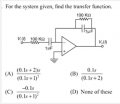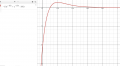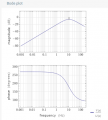# Graph of time domain Transfer function.

Joined Jan 12, 2021
66
Hello All!
I did the math and found that the transfer function of the following circuit is option C.
I later used Inverse Laplace to convert the Laplace domain Transfer function into the time domain and obtained the time domain equation. ( the time domain equation is on the left in the picture of the graph)
I graphed the time domain function and the picture of the graph is below.

My question to you guys is:
1) Is this graph showing us how the voltage at Vo(t) looks like when a certain input Vi(t) is given to the circuit ?Last edited:

#### Papabravo

Joined Feb 24, 2006
18,131
Your graph is incomplete. It should show the value -10 at t=0
As you can also see the limit at t=∞ is 0
You should also be able to find the maximum point by taking the derivative and setting it equal to 0.

Joined Jan 12, 2021
66
Your graph is incomplete. It should show the value -10 at t=0
As you can also see the limit at t=∞ is 0
You should also be able to find the maximum point by taking the derivative and setting it equal to 0.
Ty. The graph is updated.

Last edited:

#### Papabravo

Joined Feb 24, 2006
18,131
Ty. The graph is updated.
Yes, now everything makes sense. Nice job.
I don't remember clearly which response (step or impulse) this represents, but It seems likely that it should be one or the other.
The other thing to do is make a Bode plot of the magnitude and phase response so you can see how a sinusoidal input is handled.

Joined Jan 12, 2021
66
Yes, now everything makes sense. Nice job.
I don't remember clearly which response (step or impulse) this represents, but It seems likely that it should be one or the other.
The other thing to do is make a Bode plot of the magnitude and phase response so you can see how a sinusoidal input is handled.
Here is the bode plot. So what can we know from it?#### Papabravo

Joined Feb 24, 2006
18,131
We can tell that it is a reasonably narrow band pass filter with a center frequency of 10 Hz. The bandwidth is defined as the points on the magnitude plot that are 3 dB below the peak value at 10 Hz.

You can also see the -180 degree phase shift from about 270 degrees below the magnitude peak to 90 degrees above the magnitude peak. The phase shift at the magnitude peak should be -90 degrees. That would be at 180 degrees on the absolute scale.